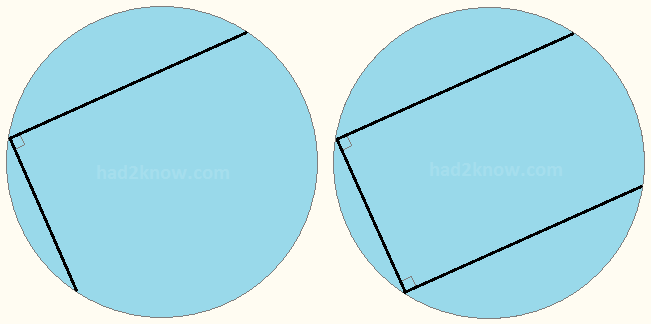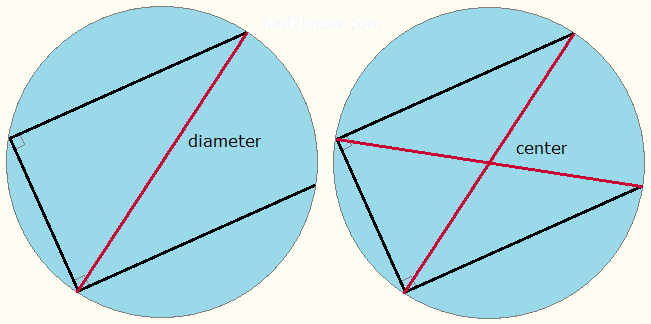# How to Find the Diameter and Exact Center of a Circle

For many kinds of do-it-yourself repair and building projects you need to find the exact center and diameter of a circular component. The geometric center of a circle is its center of gravity, while the diameter of the circle gives the widest width and allows you to compute the circumference and area.

You can use simple geometry to find two intersecting diameters of a circle. And where the two diameter lines cross is the exact center of the circle. All you need is a straight edge, a right angle, and either a pencil or string and tape to mark the lines.

Step 1: Draw a chord across the circle, somewhere away from the center.Step 2: Draw a second chord perpendicular to the first chord.

Step 3: Draw a third chord perpendicular to the second and parallel to the first.Step 4: Draw a line connecting the ends of the first and second chords. This line is the diameter of the circle.

Step 5: Draw a line connecting the ends of the second and third chords. This forms another diameter. The intersection of the two diameters is the center of the circle.### Why does this method work?

The underlying geometric principle is that whenever you circumscribe a right triangle, the hypotenuse of the triangle forms the diameter of the circle. Put another way, the circumcenter of a right triangle is the midpoint of the hypotenuse, so half the hypotenuse is the radius of the circle.

The method above works by making two perpendicular chords to form the legs of a right triangle. When you draw the third side of the triangle (the hypotenuse), it is precisely the diameter.

Also, by definition, a diameter is a line that passes through the center of a circle, so two diameters must cross at the center.